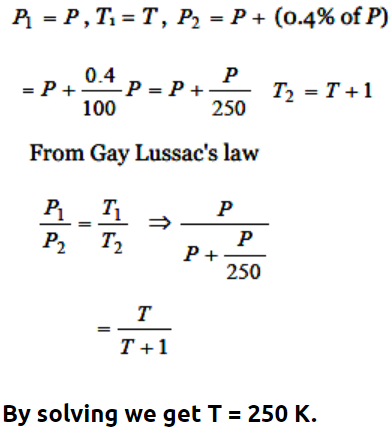## Kinetic Theory Questions and Answers Part-2

1. The mean free path of gas molecules depends on (d = molecular diameter)
a) d
b) $d^{2}$
c) $d^{-2}$
d) $d^{-1}$

Explanation: The mean free path of gas molecules depends on $d^{-2}$

2. For Boyle's law to hold the gas should be
a) Perfect and of constant mass and temperature
b) Real and of constant mass and temperature
c) Perfect and at constant temperature but variable mass
d) Real and at constant temperature but variable mass

Explanation: For Boyle's law to hold the gas should be perfect and of constant mass and temperature

3.If pressure of a gas contained in a closed vessel is increased by 0.4% when heated by 1°C, the initial temperature must be
a) 250 K
b) 250°C
c) 2500 K
d) 25°C

Explanation:4. Consider a 1 c.c. sample of air at absolute temperature $T_{0}$ at sea level and another 1 cc sample of air at a height where the pressure is one-third atmosphere. The absolute temperature T of the sample at that height is
a) Equal to $T_{0}\diagup3$
b) Equal to $3\diagup T_{0}$
c) Equal to $T_{0}$
d) Cannot be determined in terms of $T_{0}$ from the above data

Explanation: Cannot be determined in terms of $T_{0}$ from the above data

5. To double the volume of a given mass of an ideal gas at 27°C keeping the pressure constant, one must raise the temperature in degree centigrade to
a) 54°
b) 270°
c) 327°
d) 600°

Explanation:6. Which of the following statements about kinetic theory of gases is wrong
a) The molecules of a gas are in continuous random motion
b) The molecules continuously undergo inelastic collisions
c) The molecules do not interact with each other except during collisions
d) The collisions amongst the molecules are of short duration

Explanation: The molecules continuously undergo inelastic collisions

7. If a Vander-Waal's gas expands freely, then final temperature is
a) Less than the initial temperature
b) Equal to the initial temperature
c) More than the initial temperature
d) Less or more than the initial temperature depending on the nature of the gas

Explanation: If a Vander-Waal's gas expands freely, then final temperature is less than the initial temperature

8. In Vander Waal's equation $\left[P+\frac{a}{V^{2}}\right]\left(V-b\right)=RT$      the dimensions of a are
a) $M^{1}L^{5}T^{-2}$
b) $M^{0}L^{2}T^{-3}$
c) $M^{1}L^{3}T^{-2}$
d) $M^{1}L^{1}T^{-2}$

Explanation:9. At NTP, sample of equal volume of chlorine and oxygen is taken. Now ratio of No. of molecules
a) 1 : 1
b) 32 : 27
c) 2 : 1
d) 16 : 14